Function Repository Resource:

# ArgHue

Map the argument of a complex number to a color

Contributed by: Ted Ersek
 ResourceFunction["ArgHue"][arg] returns Hue[h,1,1] where h depends on the value of arg. ResourceFunction["ArgHue"][arg,abs] creates a Hue based on the argument arg and magnitude abs of a complex value.

## Details and Options

The parameter arg must be a real number.
The parameter abs must be a real number in the closed interval [0,1].
When using ResourceFunction["ArgHue"] as a ColorFunction, the setting should also be used.
ArgHue takes the following option:
 ZeroColor White approach this color as the parameter abs approaches zero
The setting of the ZeroColor option can be any color.

## Examples

### Basic Examples (2)

When arg is a real number, ArgHue[arg] returns a hue with full saturation and full brightness:

 In:=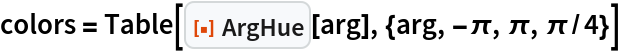Out=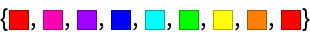Display the above colors in a way that indicates the direction in the complex plane they represent:

 In:=Out=Make a color wheel around the origin of the complex plane:

 In:=Out=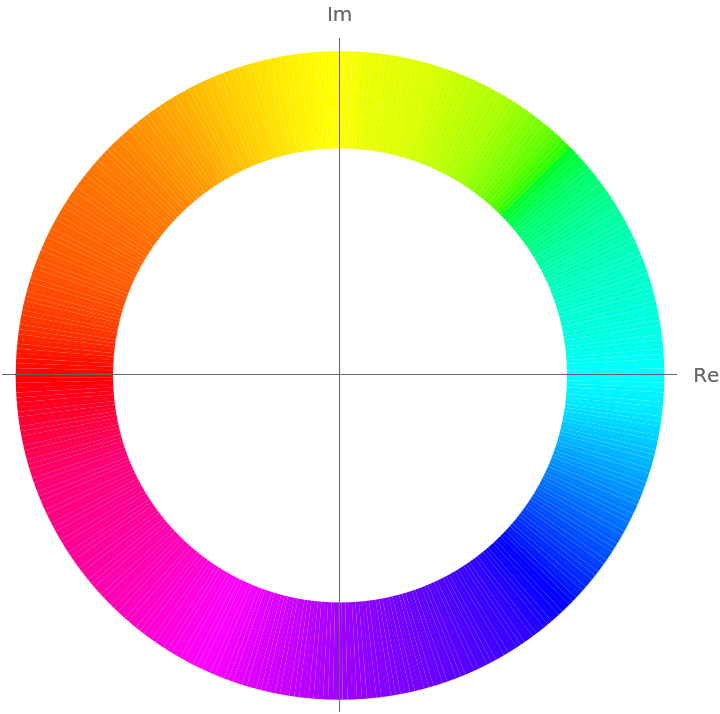### Options (5)

#### ZeroColor (5)

When the ZeroColor setting is White, ArgHue[arg,abs] returns a color that approaches White as abs approaches 0:

 In:=Out=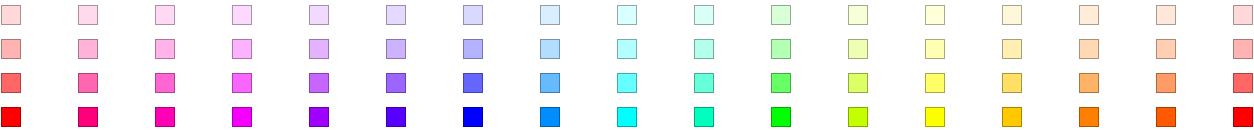When the ZeroColor setting is Black, ArgHue[arg,abs] returns a color that approaches Black as abs approaches 0:

 In:=Out=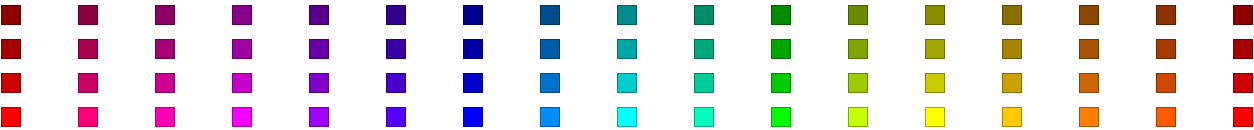Specify that White should be used where the function approaches zero:

 In:=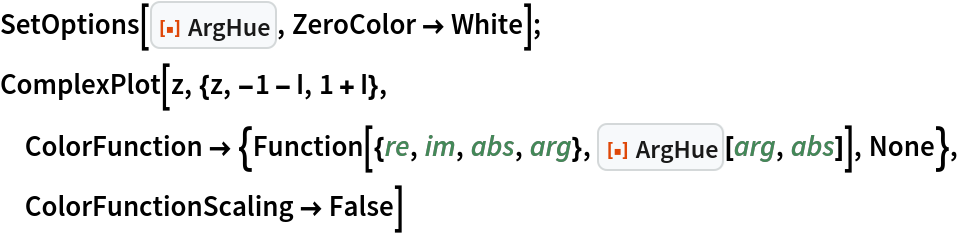Out=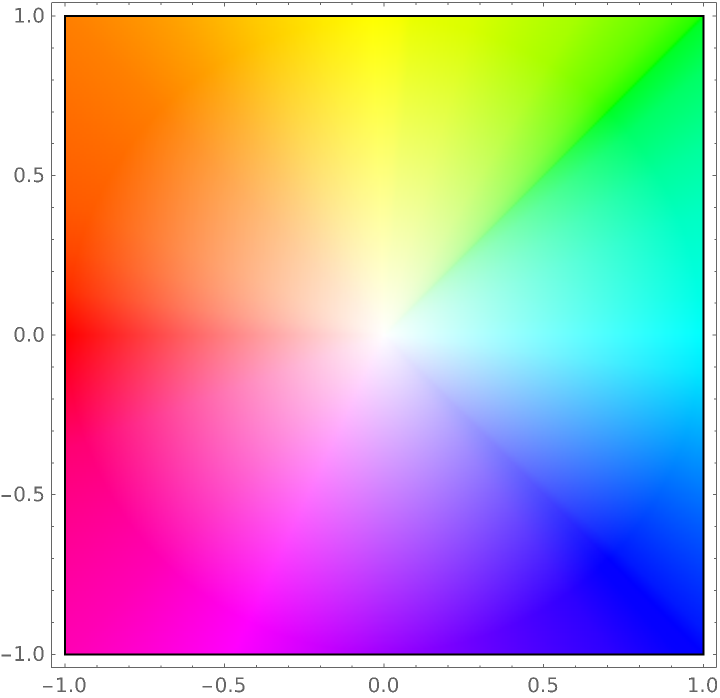Specify that GrayLevel[0.4] should be used where the function approaches zero:

 In:=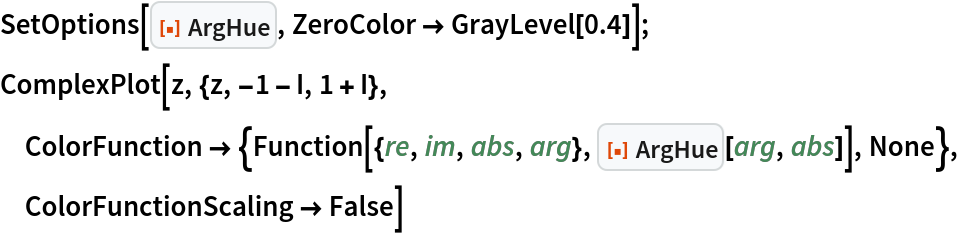Out=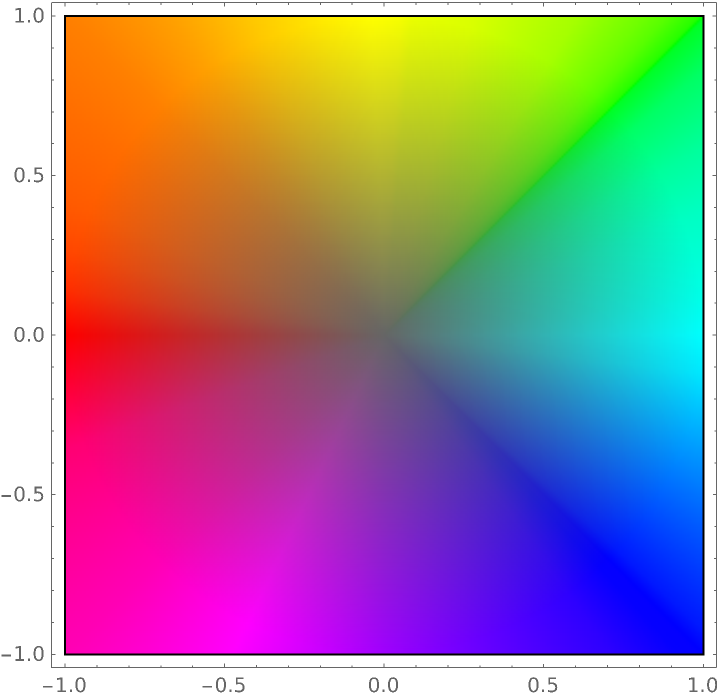Specify that RGBColor[1,0.8,0.8] should be used where the function approaches zero:

 In:=Out=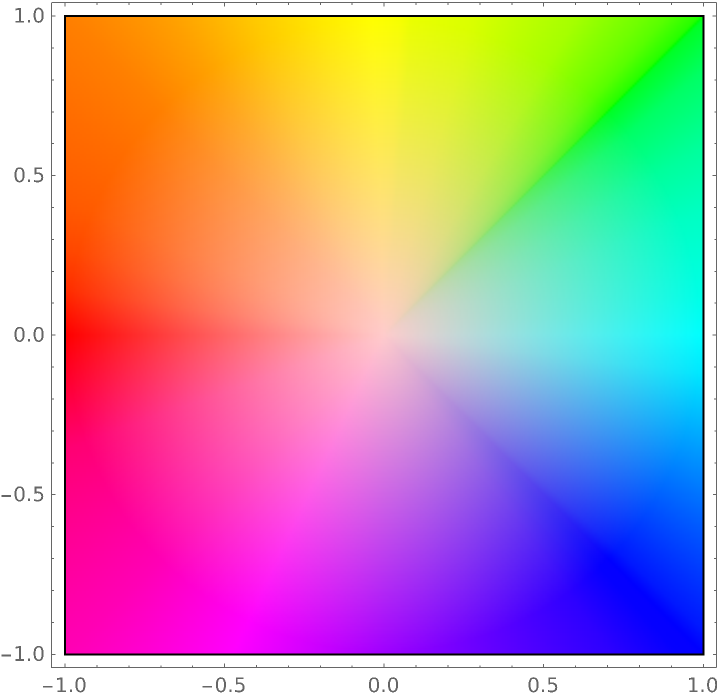### Applications (13)

#### AbsArgPlot (1)

Use ArgHue in the ColorFunction setting of AbsArgPlot:

 In:=Out=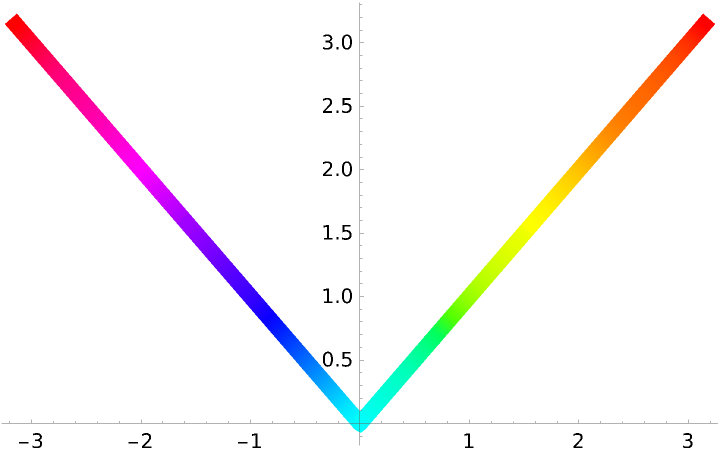#### ComplexPlot (3)

Use ArgHue in the ColorFunction setting of ComplexPlot with the hue indicating the argument of the function value:

 In:=Out=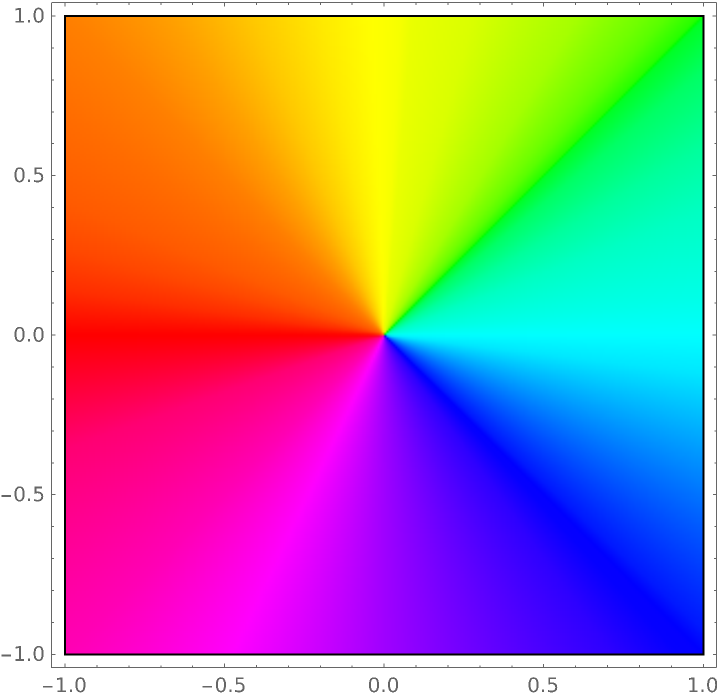Use ArgHue in the ColorFunction setting of ComplexPlot with the hue indicating the argument of the function value and a color that approaches White as the function value approaches zero:

 In:=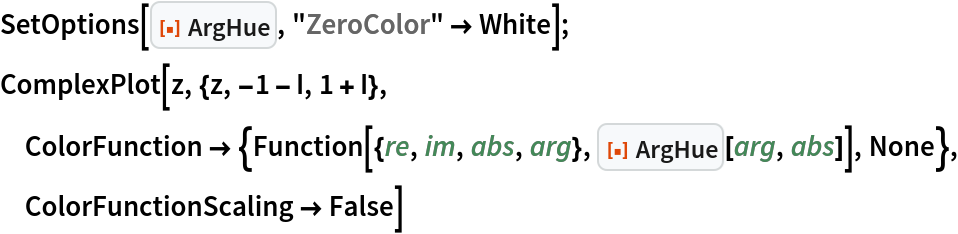Out=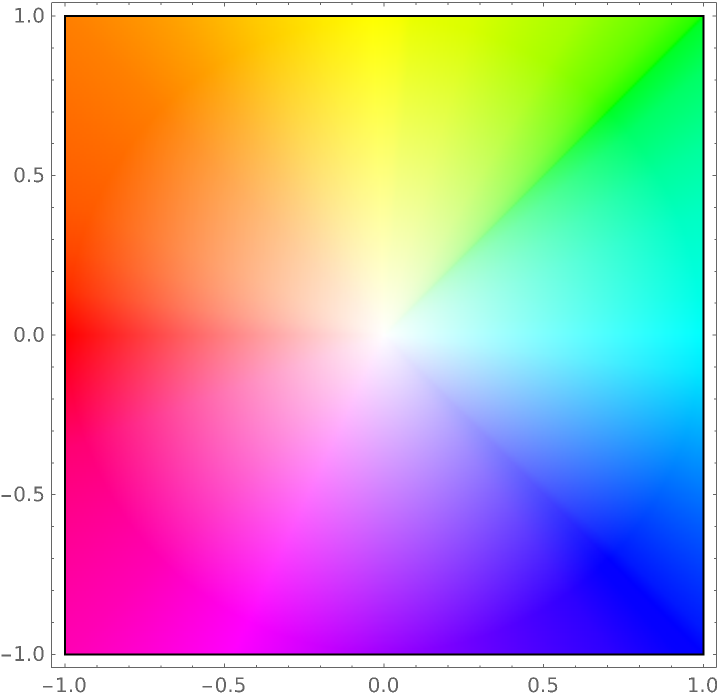Use ArgHue in the ColorFunction setting of ComplexPlot with the hue indicating the argument of the function value and a color that approaches Black as the function value approaches zero:

 In:=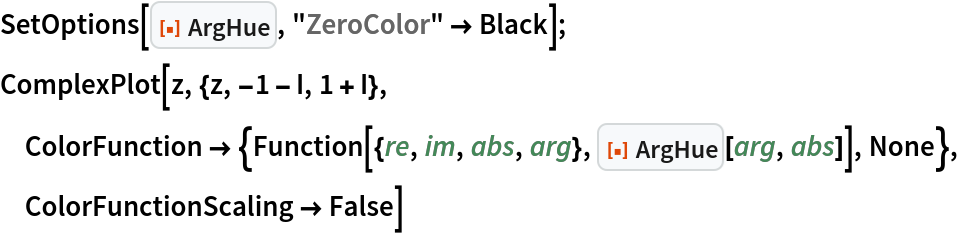Out=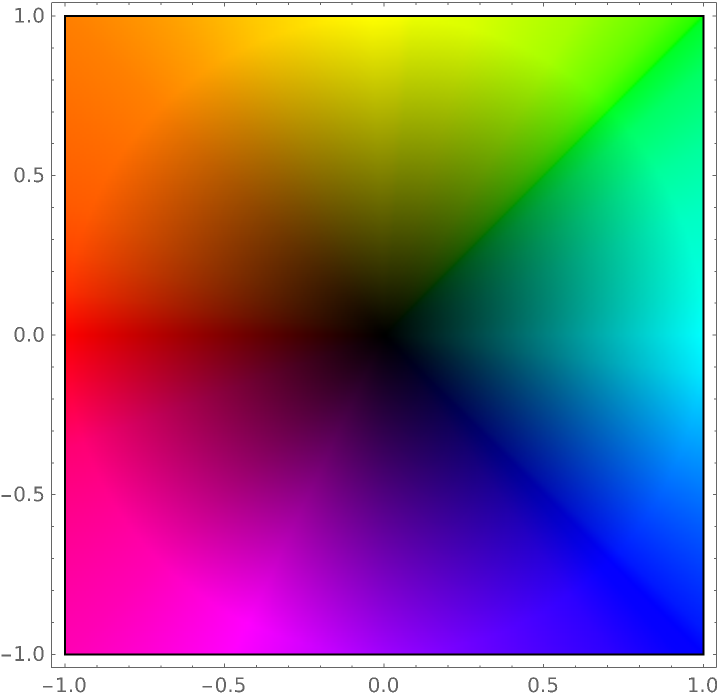#### ComplexPlot3D (3)

Use ArgHue in the ColorFunction setting of ComplexPlot3D with the hue indicating the argument of the function value:

 In:=Out=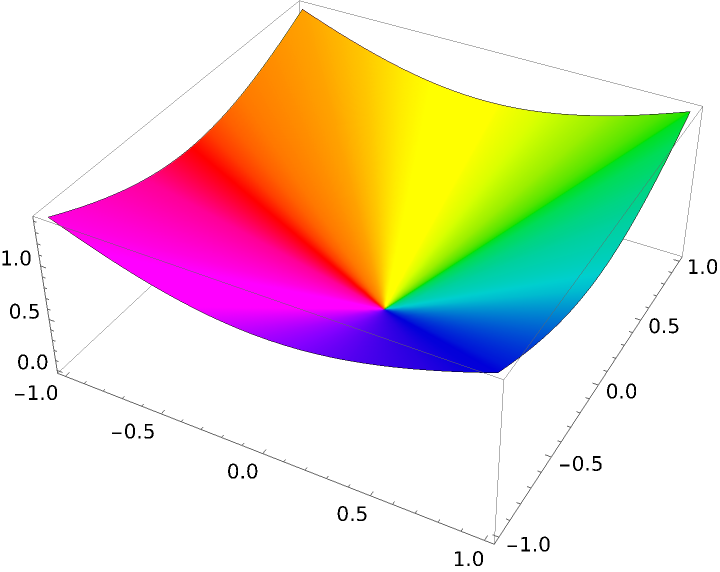Use ArgHue in the ColorFunction setting of ComplexPlot3D with the hue indicating the argument of the function value and a color that approaches White as the function value approaches zero:

 In:=Out=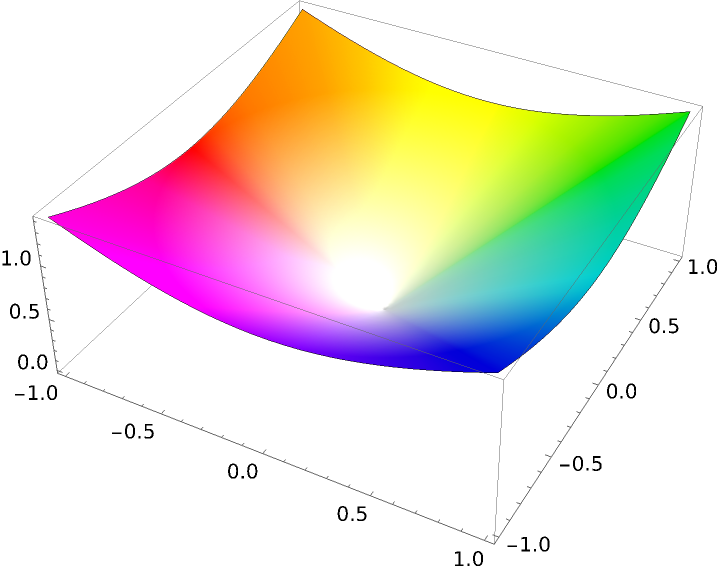Use ArgHue in the ColorFunction setting of ComplexPlot3D with the hue indicating the argument of the function value and a color that approaches Black as the function value approaches zero:

 In:=Out=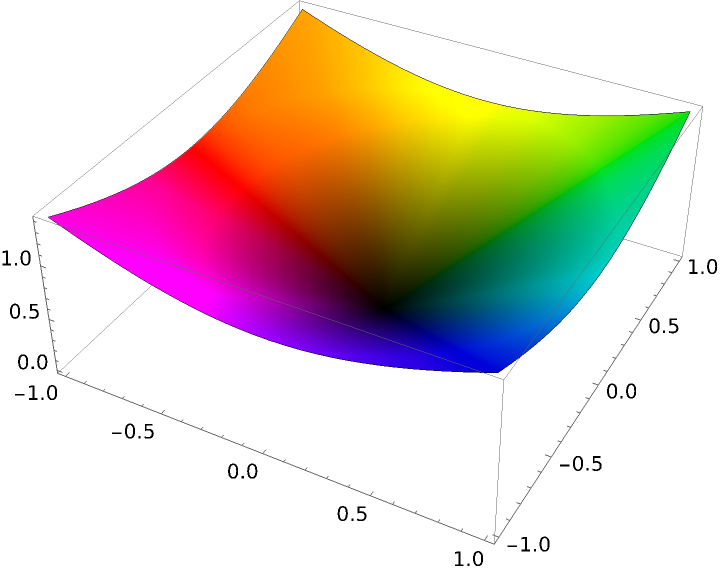#### ComplexListPlot (3)

Use ArgHue in the ColorFunction setting of ComplexListPlot with the hue indicating the argument of the function value:

 In:=Out=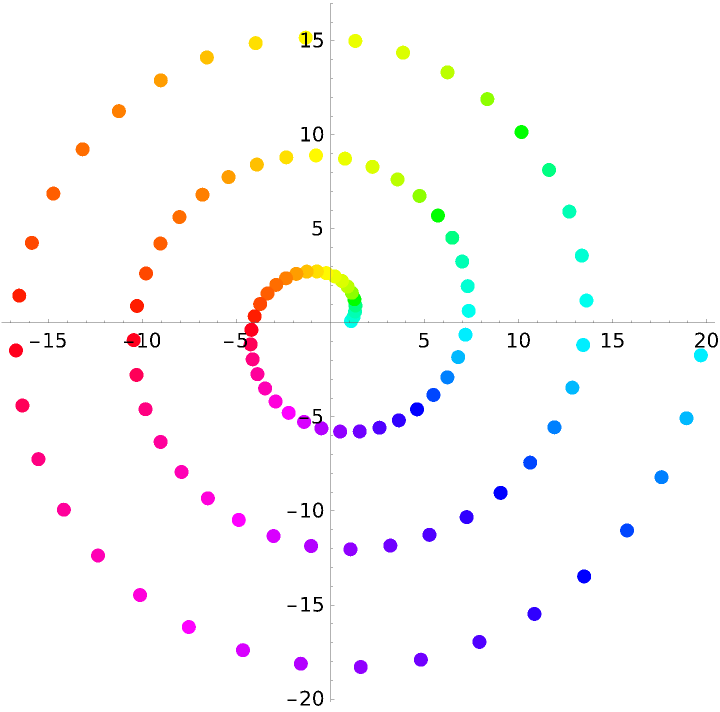Use ArgHue in the ColorFunction setting of ComplexListPlot with the hue indicating the argument of the function value and a color that approaches White as the function value approaches zero:

 In:=Out=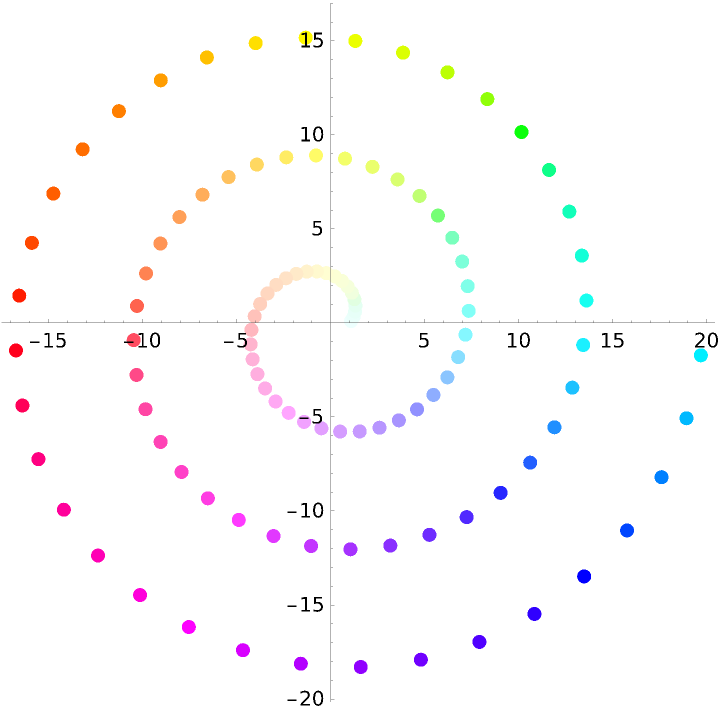Use ArgHue in the ColorFunction setting of ComplexListPlot with the hue indicating the argument of the function value and a color that approaches Black as the function value approaches zero:

 In:=Out=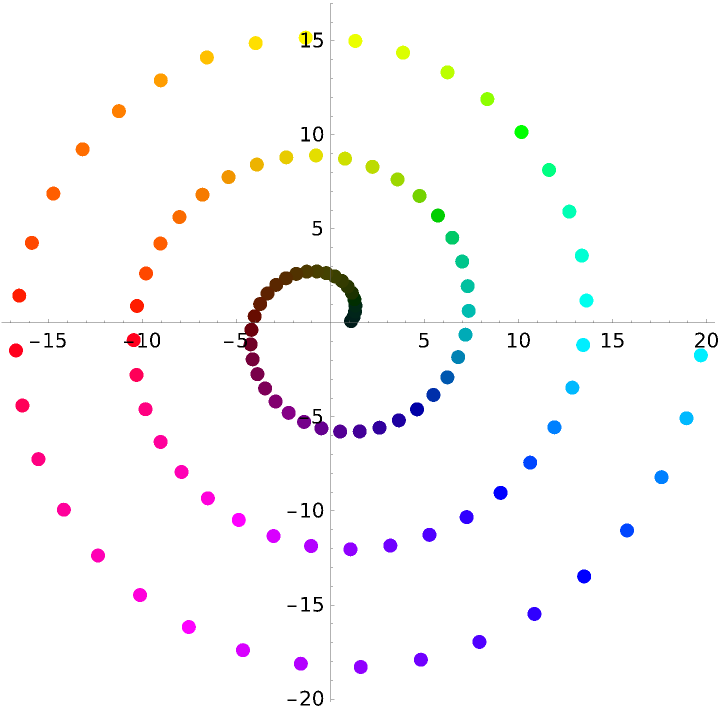#### ComplexArrayPlot (3)

Use ArgHue in the ColorFunction setting in ComplexArrayPlot with the hue indicating the argument of the function value:

 In:=Out=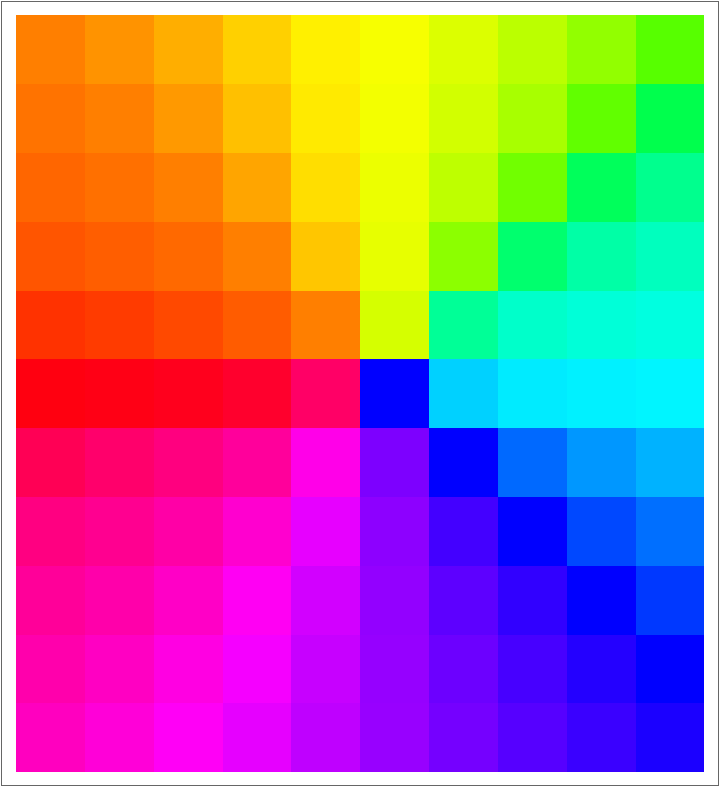Use ArgHue in the ColorFunction setting of ComplexArrayPlot with the hue indicating the argument of the function value and a color that approaches White as the function value approaches zero:

 In:=Out=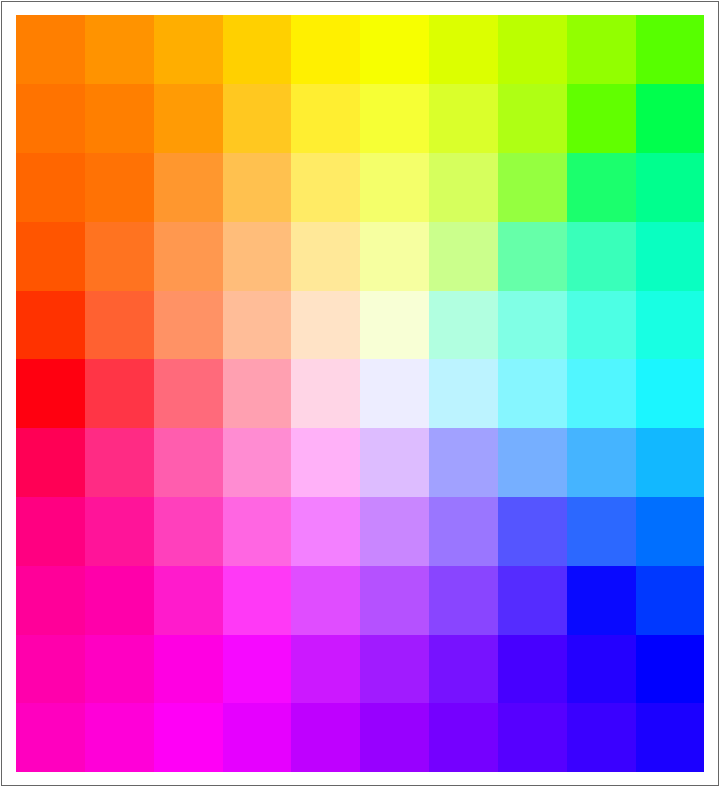Use ArgHue in the ColorFunction setting of ComplexArrayPlot with the hue indicating the argument of the function value and a color that approaches Black as the function value approaches zero:

 In:=Out=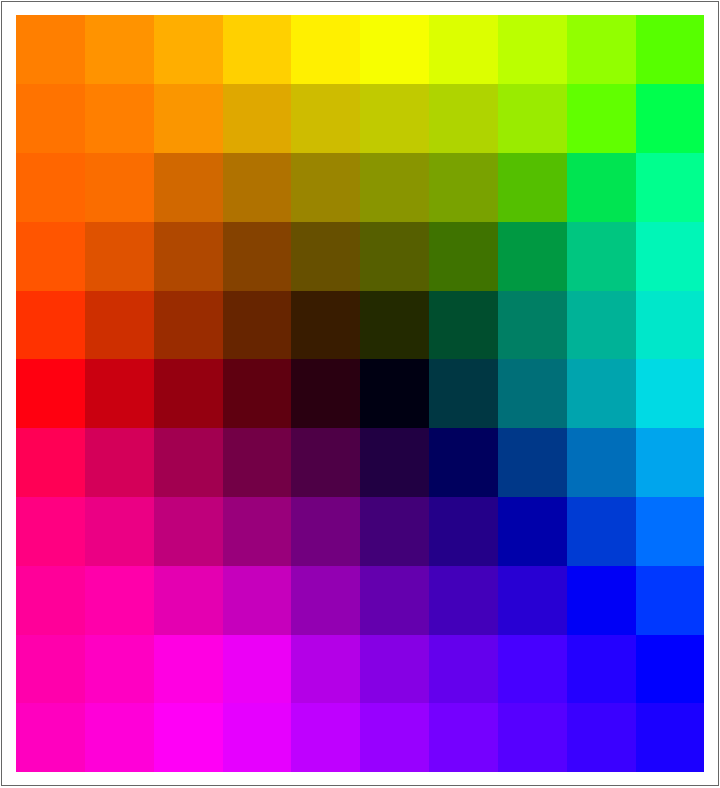### Properties and Relations (1)

ArgHue[h] is periodic with period 2π:

 In:=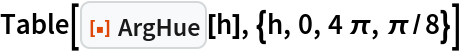Out=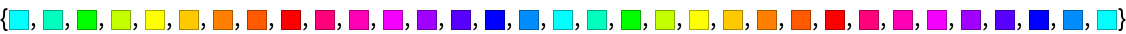Ted Ersek

## Version History

• 1.0.0 – 15 February 2023